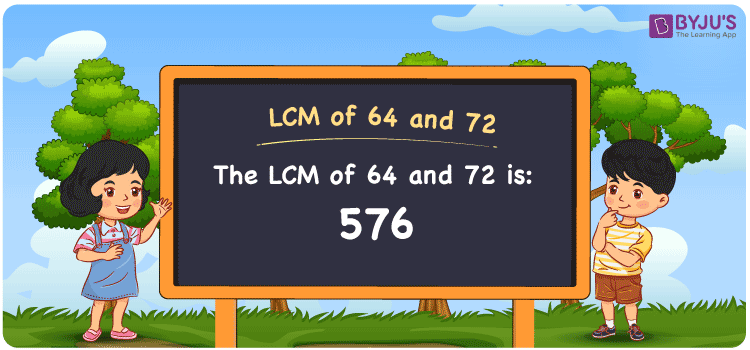Checkout JEE MAINS 2022 Question Paper Analysis : Checkout JEE MAINS 2022 Question Paper Analysis :

# LCM of 64 and 72

LCM of 64 and 72 is 576. The number which divides 64 and 72 evenly gives the LCM. The common multiples among the two numbers will help you to find the least common multiple of 64 and 72. The multiples of 64 are (64, 128, 192, 256, ….) and the multiples of 72 are (72, 144, 216, 288, 360, …..) respectively. Students can get a clear cut knowledge of the LCM concept and methods such as prime factorisation, division and listing out the multiples as per the study materials at BYJU’S.

## What is LCM of 64 and 72?

The answer to this question is 576. The LCM of 64 and 72 using various methods is shown in this article for your reference. The LCM of two non-zero integers, 64 and 72, is the smallest positive integer 576 which is divisible by both 64 and 72 with no remainder.## How to Find LCM of 64 and 72?

LCM of 64 and 72 can be found using three methods:

• Prime Factorisation
• Division method
• Listing the multiples

### LCM of 64 and 72 Using Prime Factorisation Method

The prime factorisation of 64 and 72, respectively, is given by:

64 = 2 x 2 x 2 x 2 x 2 x 2 = 2

72 = 2 x 2 x 2 x 3 x 3 = 2³ x 3²

LCM (64, 72) = 576

### LCM of 64 and 72 Using Division Method

We’ll divide the numbers (64, 72) by their prime factors to get the LCM of 64 and 72 using the division method (preferably common). The LCM of 64 and 72 is calculated by multiplying these divisors.

 2 64 72 2 32 36 2 16 18 2 8 9 2 4 9 2 2 9 3 1 9 3 1 3 x 1 1

No further division can be done.

Hence, LCM (64, 72) = 576

### LCM of 64 and 72 Using Listing the Multiples

To calculate the LCM of 64 and 72 by listing out the common multiples, list the multiples as shown below.

 Multiples of 64 Multiples of 72 64 72 128 144 192 216 256 288 320 360 384 432 448 504 512 576 576 648 640 720

LCM (64, 72) = 576

## Video Lesson on Applications of LCM## LCM of 64 and 72 Solved Examples

Determine the smallest number divisible by 64 and 72 exactly.

Solution:

The smallest number divisible by 64 and 72 exactly is LCM.

Multiples of 64 = 64, 128, 192, 256, 320, 384, ….

Multiples of 72 = 72, 144, 216, 288, 360, 432, ….

Therefore, the LCM of 64 and 72 is 576.

## Frequently Asked Questions on LCM of 64 and 72

### Find the GCF if the LCM of 64 and 72 is 576.

We know that,

LCM x GCF = 64 x 72

As LCM (64, 72) is 576

576 x GCF = 4608

GCF = 4608/576 = 8

### Find the LCM of 64 and 72.

The LCM of 64 and 72 is 576. First the multiples of 64 and 72 have to be found to get the LCM value.

Multiples of 64 = 64, 128, 192, 256, 320, 384, ……

Multiples of 72 = 72, 144, 216, 288, 360, 432, ….

The smallest multiple exactly divisible by 64 and 72 is 576.

### Show the relation between GCF and LCM of 64 and 72.

The relation between GCF and LCM of 64 and 72 is

LCM x GCF = 64 x 72

LCM x GCF = 4608